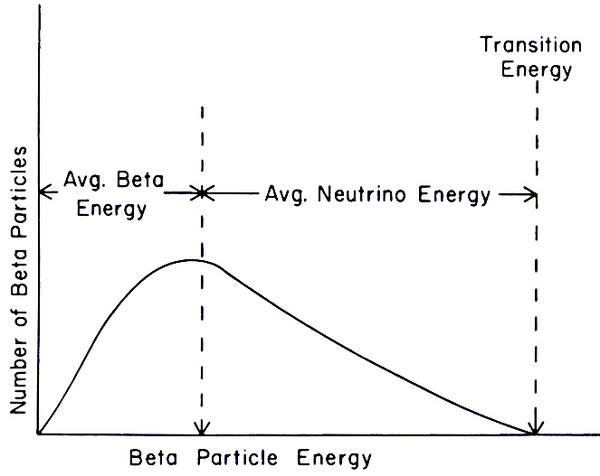Updated May 07, 2023Image: Energy spectrum of the beta particle. Author Sprawls Educational Foundation.
The average energy of the beta particle is less than the maximum energy of decay, which seems contrary to the first law of thermodynamics. To resolve this paradox, Wolfgang Pauli proposed, in 1930, that the "missing" energy was carried away by another particle, not yet discovered, which he baptized neutron, renamed neutrino in 1933 by Enrico Fermi, and observed experimentally in 1956.

### The puzzle of β radioactivity

Beta radioactivity is a type of radioactivity where an unstable atomic nucleus emits a beta particle, which can be an electron (β-) or a positron (β+).

When a nucleus is unstable, it tries to stabilize itself by getting rid of some of its excess energy. In the case of beta radioactivity, decay occurs by transforming a neutron or proton into a beta particle and into a neutrino or antineutrino.
Thus, in β- decay, a neutron transforms into a proton and an electron, so the nucleus emits an electron.
In β+ decay, a proton transforms into a neutron and a positron, so the nucleus emits a positron.

Prior to the discovery of the neutrino, scientists had observed situations where conservation of energy and momentum seemed to be violated in radioactive decay processes. For example, in beta decay, the unstable nucleus emits a beta particle and a neutrino (or antineutrino). But at that time, scientists do not know the neutrino and find that the kinetic energy of the emitted beta particle is not equal to the total energy of the decay, which violated the law of conservation of energy.
The first law of thermodynamics states that energy cannot be created or destroyed, only transformed from one form to another.
In this transformation, there was a lack of energy!
It was therefore necessary to renounce the conservation of energy but also the invariance of physical laws by translation of time. This idea was considered but it would have had profound implications for physics.
This is what has been called the beta radioactivity puzzle!
Indeed, this invariance of time is a fundamental principle of physics that underlies many theories and discoveries in physics, including Einstein's special relativity and quantum mechanics. It helps to understand the dynamics of complex physical systems and phenomena such as conservation of energy and momentum.

To solve the puzzle of β radioactivity, an Austrian theoretical physicist, Wolfgang Pauli (1900-1958) put forward the idea that decay produces not two particles but three. In this hypothesis, the laws of conservation of energy are saved.
"..., I discovered an unexpected remedy to save energy conservation laws and statistics. It is about the possibility of existence in the nuclei of neutral particles of spin, obeying the principle of exclusion, but different from photons in that they do not move at the speed of light, and that I call neutrons.". Wolfgang Pauli, Zurich, letter dated December 4, 1930 addressed to a conference taking place in Tübingen where he could not go.
Pauli suggested that his particle was very light, devoid of electrical charge, and interacted very weakly with matter, which would explain why it was difficult to detect.

In 1932, a British physicist, James Chadwick (1891-1974) discovered a real subatomic particle which had a mass similar to that of the proton, but which was neutral. Chadwick called this particle the "neutron", but this particle is completely different from the hypothetical particle proposed by Pauli.

Enrico Fermi developed the first detailed theory of beta decay using Wolfgang Pauli's hypothesis of the existence of an extra particle. It is he who gives the neutrino name "small neutron" to this particle. Fermi chose this name for the hypothetical particle Pauli proposed because he found that this particle had no electrical charge and did not easily interact with matter, making it difficult to detect. The name "neutrino" therefore reflects these properties of the particle.

It was not until 1956 that Frederick Reines (1918-1998) and Clyde Cowan (1919-1974), within the Los Alamos laboratory in the United States, experimentally discovered the existence of neutrinos, which had been proposed theoretically several decades earlier by Pauli and Fermi.

1997 © Astronoo.com − Astronomy, Astrophysics, Evolution and Ecology.